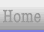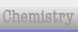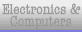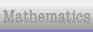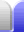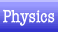# Laws of Physics

### Newton's Laws of Motion

Newton's laws of motion are three physical laws that together laid the foundation for classical mechanics. They describe the relationship between a body and the forces acting upon it, and its motion in response to said forces. They have been expressed in several different ways over nearly three centuries, and can be summarized as follows:

• First law of motion: F = 0 ⇔ a = 0 or ΣF = 0 ⇔ dv/dt = 0. When viewed in an inertial reference frame, an object either remains at rest or continues to move at a constant velocity, unless acted upon by an external force.

• Second law of motion: F = ma. The vector sum of the forces F on an object is equal to the mass m of that object multiplied by the acceleration vector a of the object. Alternatively, F = dp/dt = d(mv)/dt = mdv/dt = ma. Tthe rate of change of momentum is equal to the force applied and the change in momentum takes place in the direction of the applied force.

• Third law of motion: When one body exerts a force on a second body, the second body simultaneously exerts a force equal in magnitude and opposite in direction on the first body.

Newton's laws of motion on Wikipedia.

### Laws of Thermodynamics

The four laws of thermodynamics define fundamental physical quantities (temperature, energy, and entropy) that characterize thermodynamic systems. The laws describe how these quantities behave under various circumstances, and forbid certain phenomena (such as perpetual motion). The four laws of thermodynamics are:

• Zeroth law of thermodynamics: If two systems are in thermal equilibrium with a third system, they must be in thermal equilibrium with each other. This law helps define the notion of temperature.

• First law of thermodynamics: Because energy is conserved, the internal energy of a system changes as heat flows in or out of it. The increase in internal energy of a closed system is equal to the sum of the heat supplied to the system and the work done on the system: ΔU = Q + W. (Note that some people use a - sign for W; work done by the system rather than work done on the system.) Equivalently, machines that violate the first law (perpetual motion machines) are impossible. Heat is the flow of thermal energy from one object to another.

• Second law of thermodynamics: Heat cannot spontaneously flow from a colder location to a hotter location. The entropy of any isolated system cannot decrease. Such systems spontaneously evolve towards thermodynamic equilibrium — the state of maximum entropy of the system. Equivalently, machines that violate the second law (perpetual motion machines) are impossible.

• Third law of thermodynamics: The entropy of any pure substance in thermodynamic equilibrium approaches zero as the temperature approaches zero. The entropy of a system at absolute zero is typically zero, and in all cases is determined only by the number of different ground states it has.

Laws of thermodynamics on Wikipedia.

Thermodynamics book on Wikipedia.Home Astronomy Chemistry Electronics Mathematics Physics Field Trips Turn on javascript for email link